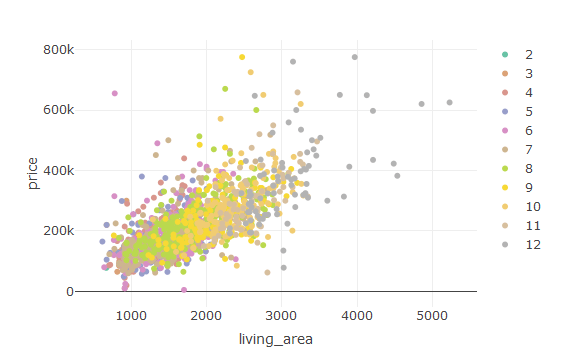0 votes
I want to explore the plotly package in R.

Can someone please help me how to build a scatter plot using RJul 6, 2018 450 views

## 1 answer to this question.

0 votes

With the help of “plotly” we can create stunning visualizations.

This is the command to create a stunning scatter plot with the help of “plotly” package.

`plot_ly(house,y=~price,x=~living_area,color=~rooms)`answered Jul 6, 2018 by
• 3,720 points

0 votes
1 answer

## How to edit the labels and limit if a plot using ggplot? - R

Add a limit to axis ticks using ...READ MORE

0 votes
1 answer

## How to join a list of data frames using map()

You can use reduce set.seed(24) r1 <- map(c(5, 10, ...READ MORE

+1 vote
1 answer

## How to convert a list of dataframes in to a single dataframe using R?

You can use the plyr function: data <- ...READ MORE

0 votes
1 answer

## How to prevent row names to be written to a file while using

Easily by: write.csv(df, "temp.csv", row.names=FALSE) READ MORE

0 votes
1 answer

## How to plot side-by-side Plots with ggplot2 in R?

By Using gridExtra library we can easily ...READ MORE

0 votes
11 answers

## Changing the legend title in ggplot

Hi, you can also try guides() to ...READ MORE

0 votes
1 answer

## How to order bars in a bar graph using ggplot2?

The key to ordering is to set ...READ MORE

0 votes
1 answer

## How to add regression line equation and R2 on graph?

Below is one solution: # GET EQUATION AND ...READ MORE

0 votes
1 answer

## How to create a box-plot using “plotly” in R?

You can use this command to create ...READ MORE

0 votes
1 answer

## How to replace NA values in a dataframe with Zero's ?

It is simple and easy: df1<-as.data.frame(matrix(sample(c(NA, 1:10), 100, ...READ MORE